#2a)There is bootstrapping bias so the center could be a bit off of the original.

#2b)Bootstrapping is with replacement. Not without.

#2c)We want original sample size not smaller

#2d) It is replacemnt from the sample, not population.

#3a)

treat=c(57,61)
control=c(42, 62,41,28)

mean(treat)-mean(control)
##  15.75

#3b

fullsamp=c(treat,control)

fullsamp
##  57 61 42 62 41 28
n=length(fullsamp)

treat1=sample(fullsamp, 2, replace = FALSE)
treat1
##  28 61
fullsamp %in% treat1
##  FALSE  TRUE FALSE FALSE FALSE  TRUE
control1=fullsamp[! fullsamp %in% treat1]

control1
##  57 42 62 41
treat1
##  28 61
mean(treat1)-mean(control1)
##  -6

#3c

d=c()

for(i in 1:20){
treatsamp=sample(fullsamp, 2, replace = FALSE)
controlsamp=fullsamp[! fullsamp %in% treatsamp]
diffmean=mean(treatsamp)-mean(controlsamp)
d=c(d, diffmean)

}

d
##     4.50  -9.00 -20.25   4.50  -5.25 -20.25  15.75  -6.00 -21.00  -9.00
##  -10.50   3.75  -5.25  -9.00   1.50  -9.00   3.75  15.75   5.25   5.25
{hist(d, breaks = 20)
abline(v=mean(treat)-mean(control), col="blue")}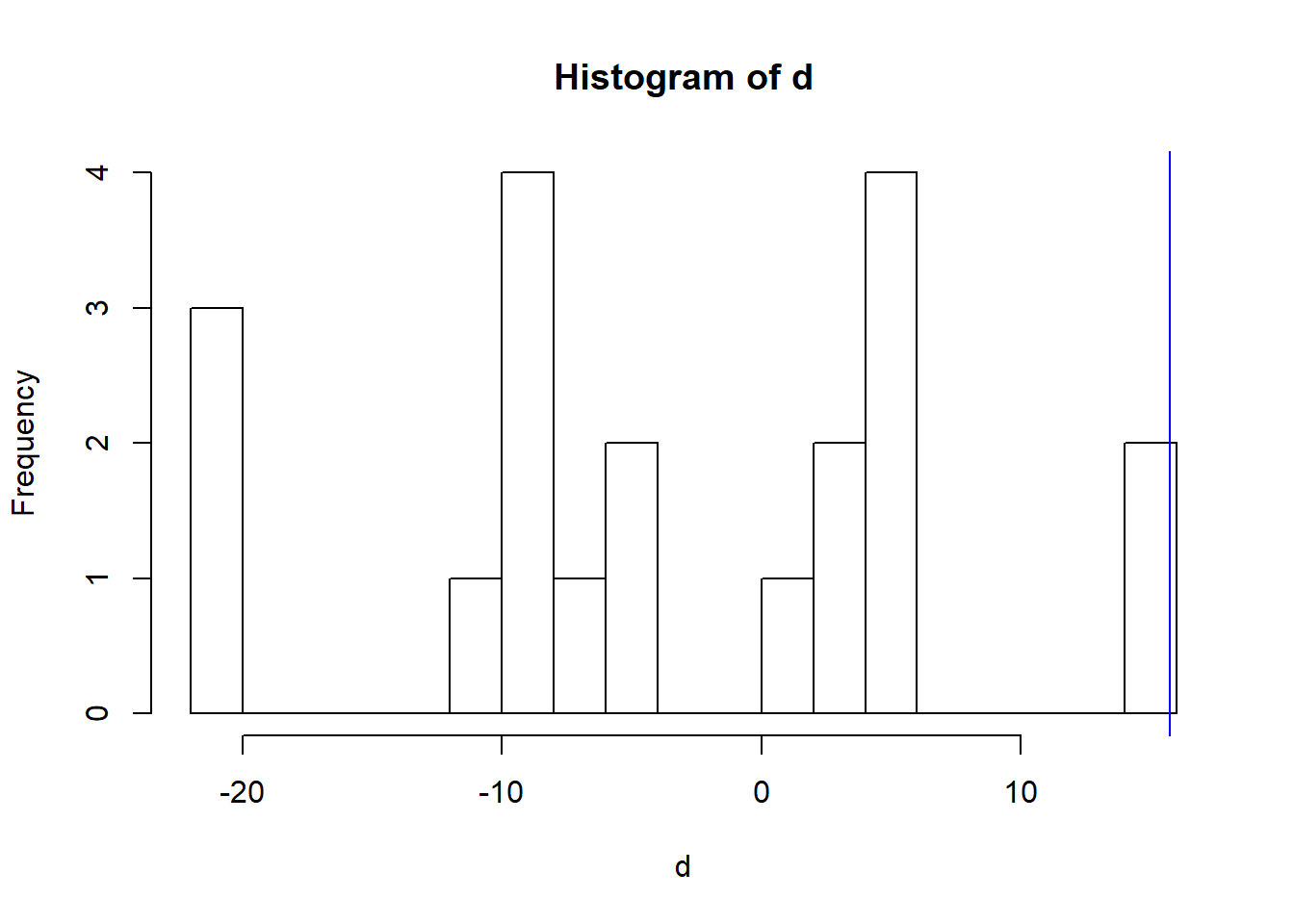#3d

pvalue=5/20=.25

#3e 3/15=.2. My estimated p value wasn’t too far off at .25.

#4a

calls<-read.csv("calls80.csv",

callvec=c(calls$length) hist(callvec)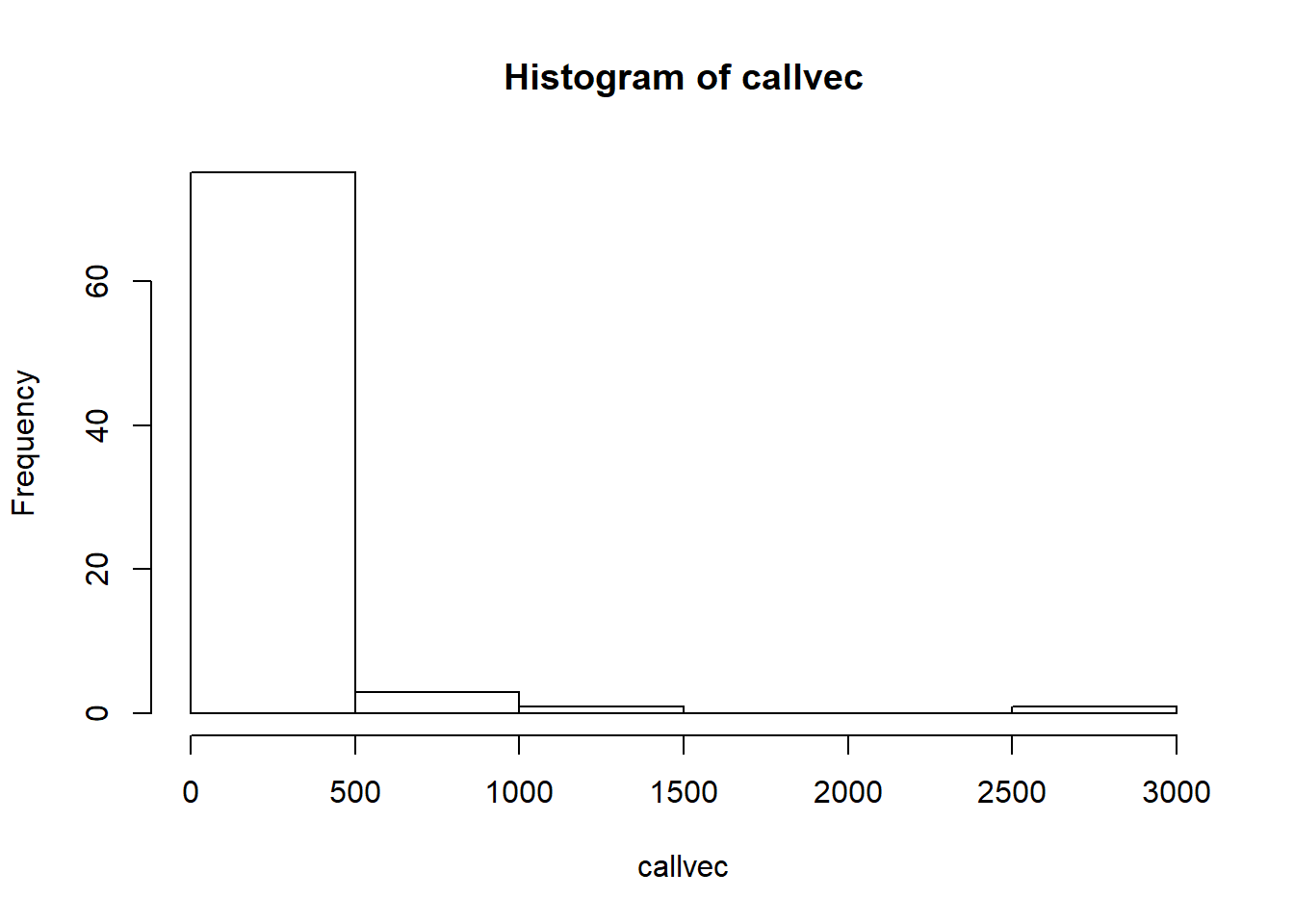#4b bootStrapCI<-function(data, nsim){ n<-length(data) bootCI<-c() for(i in 1:nsim){ bootSamp<-sample(1:n, n, replace=TRUE) thisXbar<-mean(data[bootSamp]) bootCI<-c(bootCI, thisXbar) } return(bootCI) } callsBootCI=bootStrapCI(callvec, 1000) hist(callsBootCI)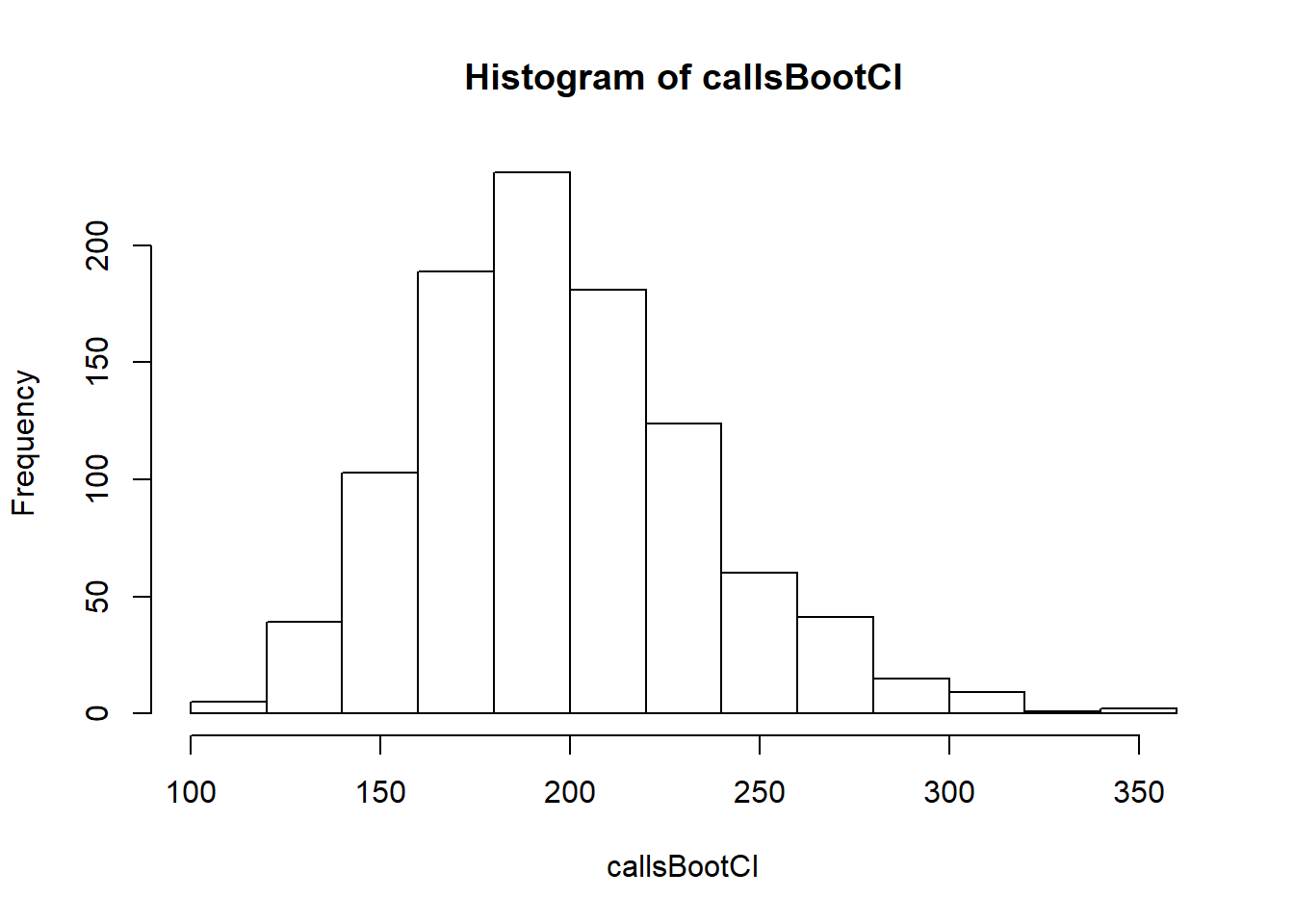#4c The right tail is decreases less rapidly than the left so it isnt symetrical like in a normal curve. That means that the quantiles on either side are not the same standard deviaions away from the center. #4d smallcall=c(104, 102, 35, 211, 56, 325, 67, 9, 179, 59) smallcallbootCI=bootStrapCI(smallcall, 1000) hist(smallcall)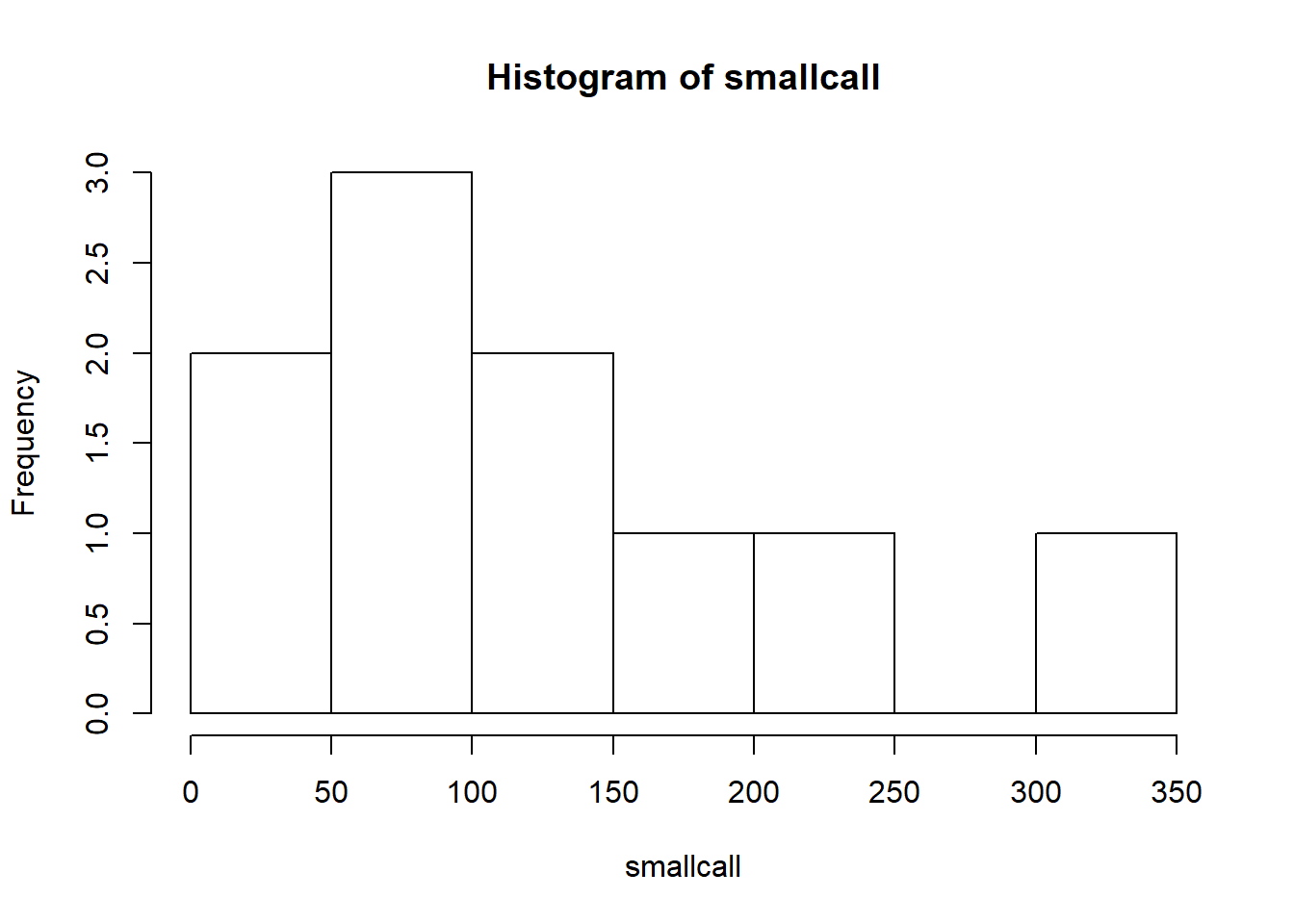It is further from the normal #4e The standard error is larger for the smaller sample because with less overall data, it will be less accurate. A sample of the poulation already represents trends with some error, and this is now a sample of the sample of the population. #5a trees<-read.csv("nspines.csv", header=TRUE) View(trees) northtrees=c(trees$dbh[1:30])

southtrees=c(trees\$dbh[31:60])

boxplot(northtrees, southtrees, names=c("northtrees", "southtrees"), col="green")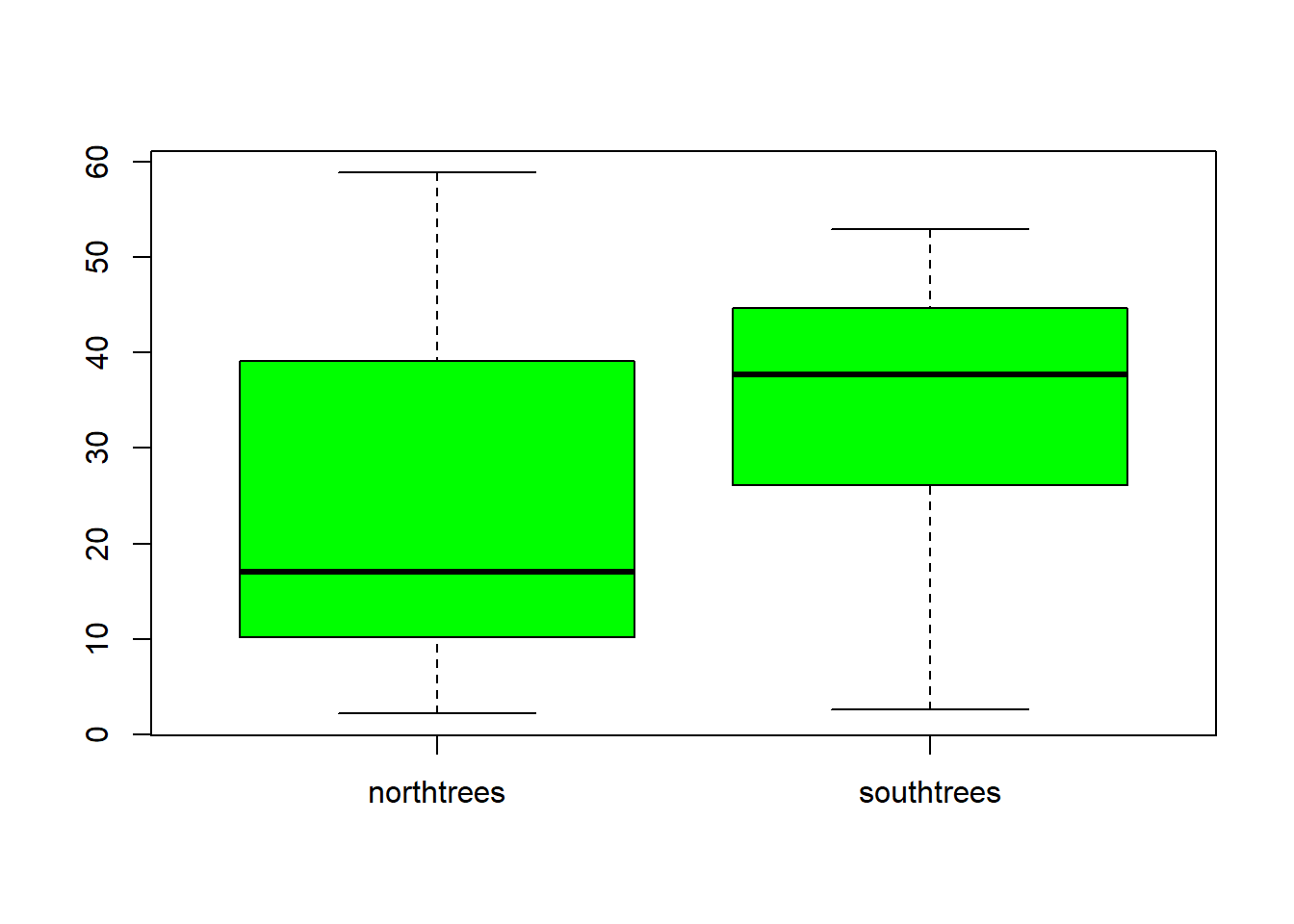There is 30 of each data so it seems reasonble to use t procedures.

#5b

mean(northtrees)-mean(southtrees)
##  -10.83333

#5c

bootStrapCI2<-function(data1, data2, nsim){

n1<-length(data1)
n2<-length(data2)

bootCI2<-c()

for(i in 1:nsim){
bootSamp1<-sample(1:n1, n1, replace=TRUE)
bootSamp2<-sample(1:n2, n2, replace=TRUE)
thisXbar<-mean(data1[bootSamp1])-mean(data2[bootSamp2])
bootCI2<-c(bootCI2, thisXbar)
}

return(bootCI2)
}

treesBoot=bootStrapCI2(northtrees, southtrees, 1000)

hist(treesBoot)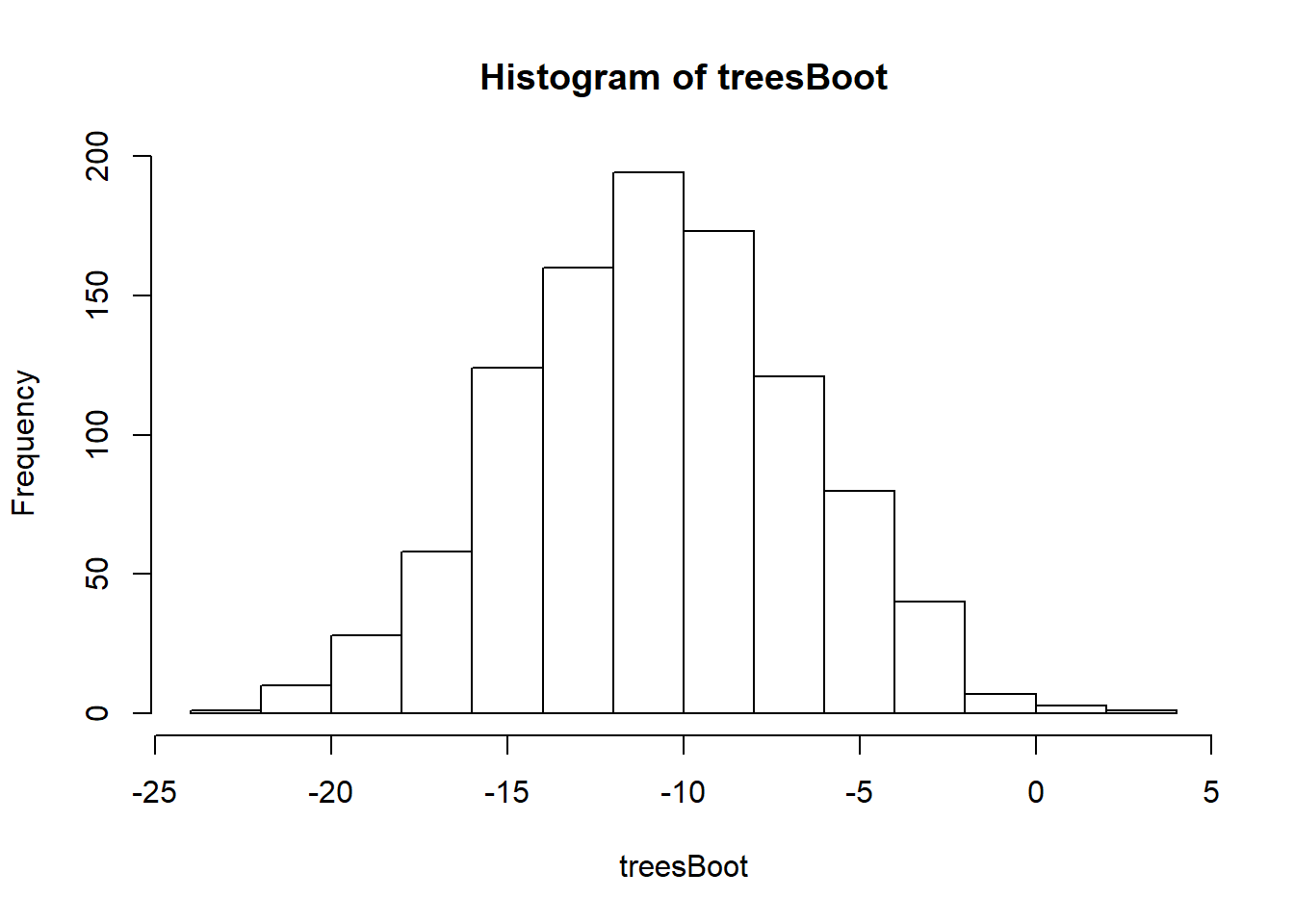#5d

quantile(treesBoot, c(0.025, 0.975))
##      2.5%     97.5%
## -18.42450  -2.96325
boottreeSE<-sd(treesBoot)
(mean(northtrees)-mean(southtrees))+c(-1,1)*qt(0.975, df=14)*boottreeSE
##  -19.600598  -2.066068

#5e The bootstrap distribution appears to be approximatly normal and centerd around the observed statistic so the hybrid method seems to be reliable.

#5f

t.test(northtrees, southtrees)
##
##  Welch Two Sample t-test
##
## data:  northtrees and southtrees
## t = -2.6286, df = 55.725, p-value = 0.01106
## alternative hypothesis: true difference in means is not equal to 0
## 95 percent confidence interval:
##  -19.090199  -2.576468
## sample estimates:
## mean of x mean of y
##  23.70000  34.53333

The bootstrapping intervals were different by about .5, higher on the left and lower on the right. I would use the bootstrapped interval because it simulated multiple samples.